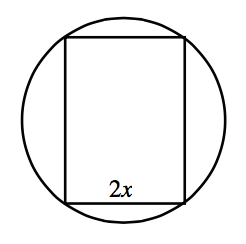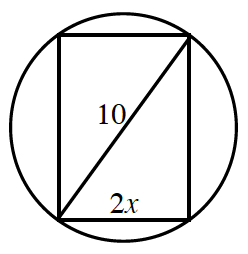### Home > PC3 > Chapter 3 > Lesson 3.1.1 > Problem3-15

3-15.The circle at right has a diameter of $10$ cm. The figure inscribed in the circle is a rectangle with width $2x$. Express each of the following quantities in terms of $x$.1. The perimeter of the rectangle.

Write an expression for the height of the rectangle.
$(2x)^2+(h)^2=(10)^2$

2. The area of the rectangle.

Multiply the expressions for the base and height.